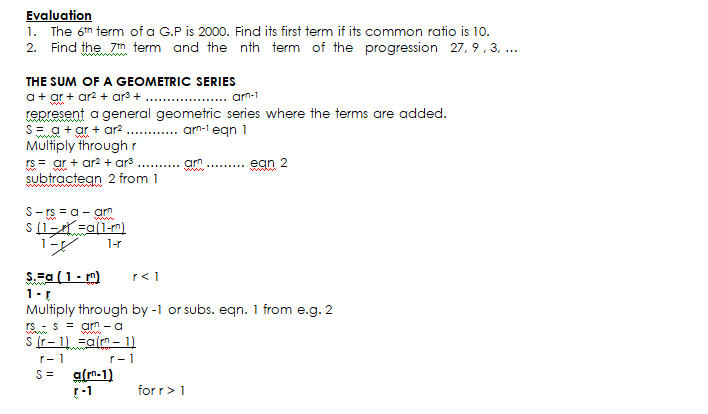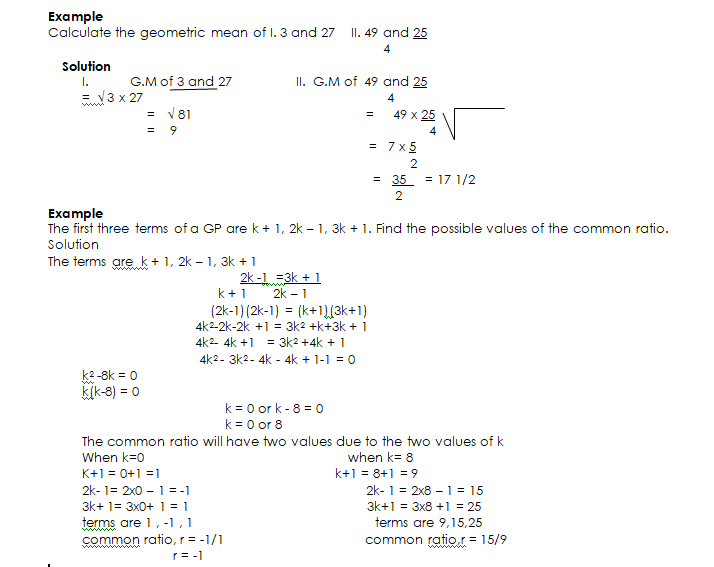# Geometric Progressions

Welcome to class!

In today’s class, we will be talking about geometric progressions. Enjoy the class!

### Geometric Progressions#### Definition of G.P

The sequence 5, 10, 20, 40 has a first term of 5 and the common ratio

Between the term is 2 e.g. (10/5 or 40/2o = 2).

A sequence in which the terms either increase or decrease in a common ratio is called a Geometric Progression

(G. P)

1. P: a, ar,     ar2,        ar3 ………………

Denotations in G. P

a    = 1st term

r    = common ratio

Un = nth term

Sn  = sum

##### The nth term of a G. P

The nth term = Un

Un = arn-1

1st term   = a

2nd term = a x r =ar

3rd term = a x r x r = ar2

4th term = a x r x r x r =  ar3

8thterm  =  a x r x r x r x r x r x r x r  =  ar7

nth term  =  a x r x r x r x ……….. arn-1###### General Evaluation
1. In the 2nd and 4th term of a G.P are 8 and 32 respectively, what is the sum of the first four terms. (a) 28 (b)  40    (c)  48    (d) 60
2. The sum of the first five terms of the G.P. 2, 6, 18, is (a) 484 (b) 243      (c) 242    (d) 130
3. The 4th term of a GP is -2/3 and its first term is 18 what is its common ratio. (a) ½    (b) 1/3 (c) -1/3   (d)  -1/2
4. If the 2nd and 5th term of a G. P. are -6 and 48 respectively, find the sum of the first four terms: (a) -45 (b) -15     (c) 15    (d) 33
5. Find the first term of the G.P. if its common ratio and sum to infinity – 3/3 and respectively (a) 48 (b) 18 (c) 40   (d) -42

In our next class, we will be talking about the Quadratic Equation.  We hope you enjoyed the class.

Should you have any further question, feel free to ask in the comment section below and trust us to respond as soon as possible.

How Can We Make ClassNotesNG Better? - CLICK to Tell Us Now!